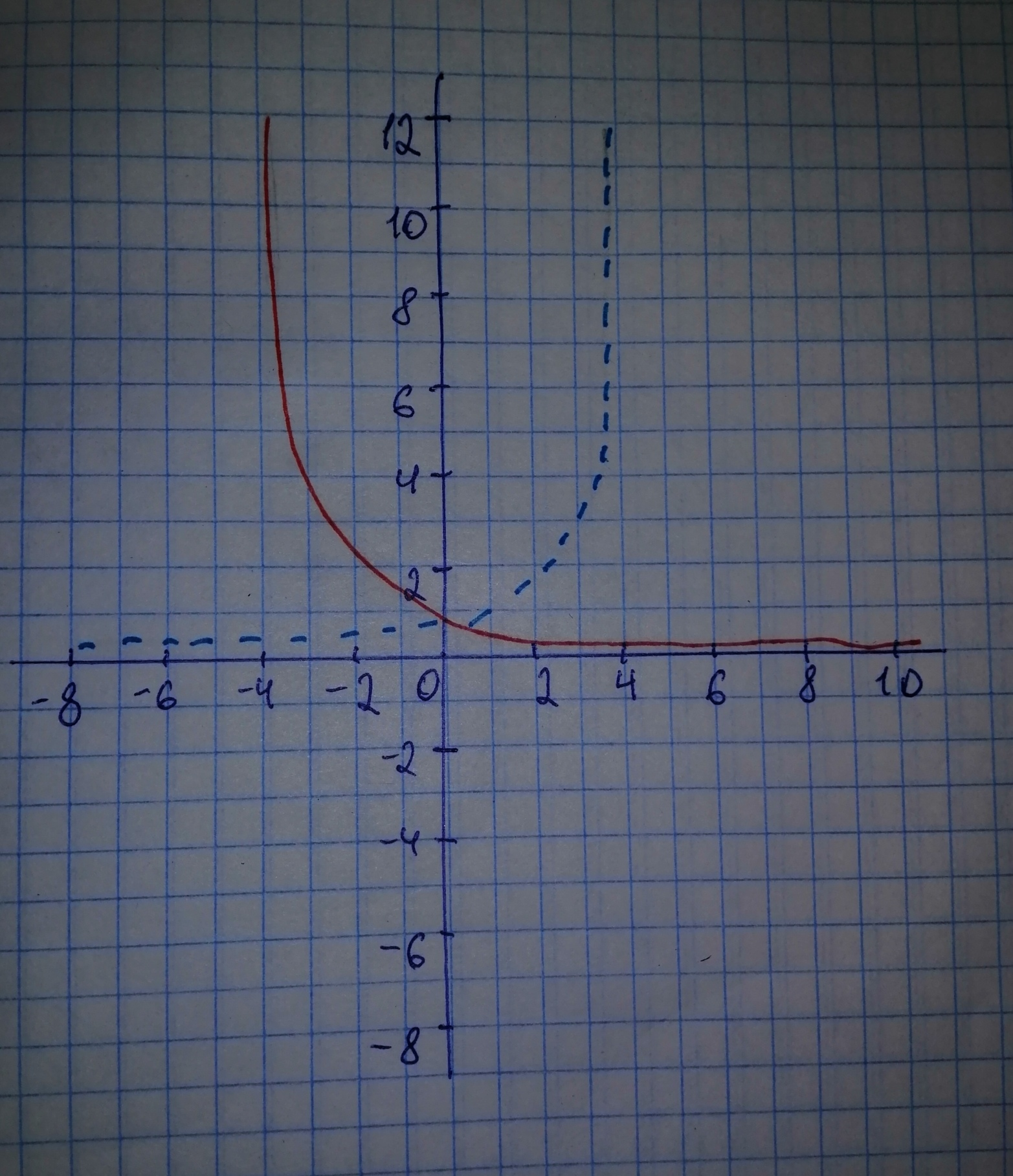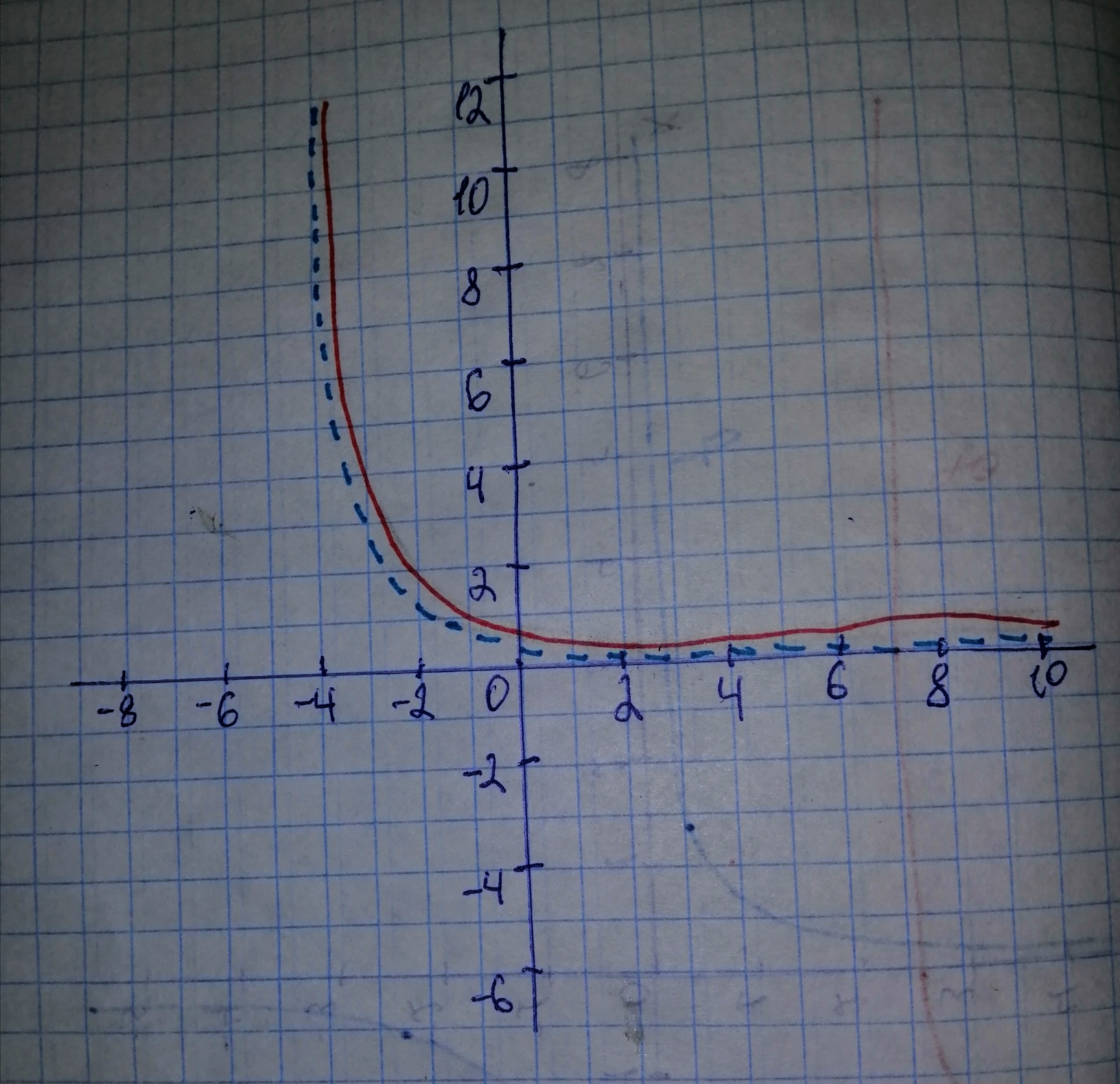jernplate8

2020-11-06

Use transformations to sketch the graph of the function.Nathaniel Kramer

Step 1

Remember that: When we reflect the graph of $y=f\left(x\right)$ in the y-axis, we get the graph for $y=f\left(-x\right)$
We will start the graph of the standard function $y={2}^{x}$ (represented by blue dashed curve)
Reflect the graph of $y={2}^{x}$ in the y-axis, to get the graph for $y={2}^{-x}$ (represented by black solid curve)Step 2
Remember that: When we shift the graph of $y=f\left(x\right)$ by k units upwards, we get the graph for
Shift the graph of $y={2}^{-x}$ by 1 unit upwards, to get the graph for (represented by red solid curve).
The graph for $y={2}^{-x}$ is represented by black dashed curve.Do you have a similar question?